METASOFT/ForestPMPlot

METASOFT is a free, open-source meta-analysis software tool for genome-wide association study analysis, designed to perform a range of basic and advanced meta-analytic methods in an efficient manner.
ForestPMPlot is a free, open-source a python-interfaced R package tool for analyzing the heterogeneous studies in meta-analysis by visualizing the effect size differences between studies. The resulting plot can facilitate the better understanding of heterogeneous genetic effects on the phenotype in different study conditions.

Overview

METASOFT provides the following methods:
Fixed Effects model (FE)
Fixed effects model based on inverse-variance-weighted effect size.
Random Effects model (RE)
Conventional random effects model based on inverse-variance-weighted effect size (very conservative).
Han and Eskin's Random Effects model (RE2)
New random effects model optimized to detect associations under heterogeneity. (Han and Eskin, AJHG 2011)
Binary Effects model (BE)
New random effects model optimized to detect associations when some studies have an effect and some studies do not. (Han and Eskin, PLoS Genetics 2012)
METASOFT provides the following estimates:
Summary effect size estimates
Beta and standard errors for both fixed effects model and random effects model.
Heterogeneity estimates
Cochran's Q statistic and its p-value, and I2
M-values
Posterior probability that an effect exists in each study. (Han and Eskin, PLoS Genetics 2012)

ForestPMPlot is a visualization tool. The resulting plot consists of the following components:

Forest Plot
For each study, it displays p-value, study name, log odds ratio and its standard error and summary statistic.
m-value
m-value is the posterior probability that the effect exists in each study. m-values have the following interpretations.
• Small m-value (e.g. < 0.1): The study is predicted to not have an effect.

• Large m-value (e.g. > 0.9): The study is predicted to have an effect.

• Otherwise: It is ambiguous to make a prediction.

m-value is estimated utilizing cross-study information (Han and Eskin, 2012 Link).
PM Plot
PM-Plot visualizes the m-value of each study along with its p-value. The X-axis of the PM-Plot represents the m-value between 0 and 1 and the Y-axis represents the statistical significance, −log10(p-value). PM-Plot allows us to predict which study have an effect and which study does not have an effect. The dot size represents the study sample size.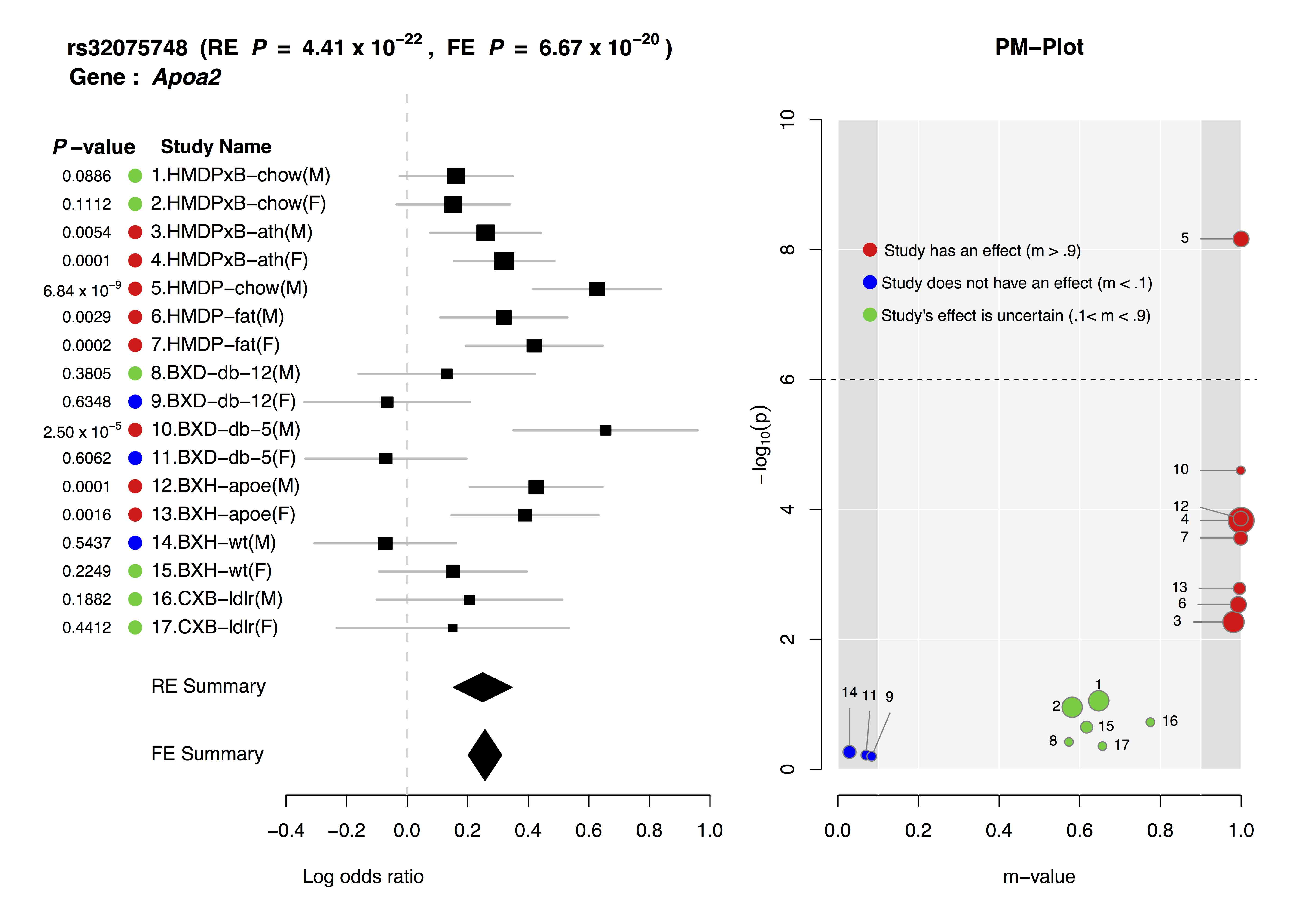• Metasoft.zip containing the followings,

• Metasoft.jar (java archive package file)

• HanEskinPvalueTable.txt (tabulated p-value file required to perform RE2)

• example.txt (example meta-analysis data consisting of 3 studies)

• plink2metasoft_subroutine.R (a subroutine file used by format converter)

• ForestPMPlot.zip containing the followings,

• pmplot.py (main python script)

• forestpmplot.R (R ploting engine)

• data/input.txt (a sample MetaSoft input file )

• data/output.txt (a sample MetaSoft output file )

• study.name.txt (a sample file containing a list of study names)

• study.order.txt (a sample file containing a list of display indices )

• run.sh (a sample running script for generating Apoa2.pdf )

• Apoa2.pdf (a sample resulting ForestPMPlot )

You can SUBSCRIBE to our mailing list to be notified for any major updates and bug fixes.

Version/bug info

METASOFT

• v2.0.0 (2012-02-15) M-values are computed using exact calculation. User interface has been changed.

• v1.0.0 (2011-08-25) First major release. Bug fix.

• v0.3.1 (2011-07-07) Bug fix.

• v0.3.0 (2011-07-06) Bug fix. Binary effects model and M-values implemented.

• v0.2.2 (2011-05-19) Bug fix.

• v0.2.1 (2011-05-16) Small changes in output format.

• v0.2.0 (2011-05-10) Bug fix. Genomic control method implemented.

• v0.1.0 (2011-04-18) Initial prototype version deployed

ForestPMPlot

• v1.0.3 (2015-05-13) Bug fix.

• v1.0.2 (2015-03-16) Bug fix.

• v1.0.1 (2014-10-24) Bug fix.

• v1.0.0 (2014-08-12) First major release.

User's guide

METASOFT

Important: User interface has been changed since v2.0.

• Usage:

usage: java -jar Metasoft.jar [options]
-input <FILE>                       Input file (Required)
-output <FILE>                      Output file (default='out')
-log <FILE>                         Log file (default='log')
-pvalue_table <FILE>                Pvalue table file (default='HanEskinPvalueTable.txt')
-lambda_mean <FLOAT>                (Random Effects) User-specified lambda for mean effect part
(default=1.0)
-lambda_hetero <FLOAT>              (Random Effects) User-specified lambda for heterogeneity
part (default=1.0)
-binary_effects                     Compute binary effects model p-value (default=false)
-binary_effects_sample <INT>        (Binary effects) Number of importance sampling samples
(default=1,000)
-binary_effects_p_thres <FLOAT>     (Binary effects) P-value threshold determining if we will
use large number of samples (default=1E-4)
-binary_effects_large <INT>         (Binary effects) Large number of importance sampling samples
for p-values above threshold (default=100,000)
-mvalue                             Compute m-value (default=false)
-mvalue_method <METHOD_NAME>        Which method to use to calculate m-value between 'exact' and
'mcmc' (default=exact)
-mvalue_p_thres <FLOAT>             Compute m-values only for SNPs whose FE or RE2 p-value is
below this threshold (default=1E-7)
-mvalue_prior_beta <ALPHA BETA>     Alpha and Beta value for Beta dist prior
(default=1.0,1.0)
-mvalue_prior_sigma <FLOAT>         Sigma value for normal prior N(0, sigma^2) for effect
(default=0.2)
-mcmc_sample <INT>                  (MCMC) Number of samples (default=10,000)
-mcmc_burnin <INT>                  (MCMC) Number of burn-in (default=1,000)
-mcmc_max_num_flip <INT or FLOAT>   (MCMC) Usual move is flipping N bits where N ~
U(1,max_num_flip). If an integer value i >= 1 is given,
max_num_flip = i. If a float value 0 < k < 1 is given,
max_num_flip = k * #studies. (default=0.1)
-mcmc_prob_random_move <FLOAT>      (MCMC) Probability that a complete randomization move is
suggested (default=0.01)
-seed <INT>                         Random number generator seed (default=0)
-verbose                            Print RSID verbosely per every 1,000 SNPs (default=false)
-help                               Print help
Binary Effects Model

BE is another kind of random effects model assuming that the effects either exists or not in the studies. Our simulations show that BE is often more powerful than RE2. However, BE requires importance sampling to calculate p-value and is slower than RE2. To use BE, use option -binary_effects. We implemented an adaptive sampling that performs BE with smaller number of samples first (-binary_effects_sample option), and if the calculated p-value exceeds a threshold (-binary_effects_p_thres option), then a larger number of samples are used for higher accuracy (-binary_effects_large option).

M-value

To compute m-values, use option -mvalue. M-values require two priors: Beta distribution prior for the presence/absence of effect (specified by -mvalue_prior_beta option), and normal distribution prior for the effect size (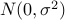where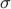is specified by -mvalue_prior_sigma option). The default for Beta distribution prior is uniform distribution (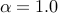and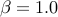) and the default foris 0.2. Since we assume a normal prior for effect size, for quantitative traits, the value ofhas to be chosen carefully considering the unit of the data. One can choose a value based on the data in an empirical Bayes style, or a rough and ad-hoc approach is just using the default value (0.2) designed for dichotomous traits.

The computation time is exponential to the number of studies. If the number of studies is small (e.g. <10), an exact calculation is feasible (-mvalue_method exact option). If the number of studies is large, MCMC should be used (-mvalue_method mcmc option). The user can specify the number of burnin (-mcmc_burnin) and the number of samples (-mcmc_sample). The MCMC move is flipping the existence of effect for N studies where N is sampled from Uniform(1, max_num_flip) where max_num_flip is specified with -mcmc_max_num_flip option.

• Input File format:
Rows are SNPs.
1st column is RSID.
2nd and 3rd columns are effect size (beta) and its standard error of study 1.
4th and 5th columns are effect size (beta) and its standard error of study 2.
6th and 7th columns are effect size (beta) and its standard error of study 3.
and so on…
Please write “NA” for missing beta and its standard error.

rsAAAAAA study1beta study1stderr study2beta study2stderr study3beta study3stderr
rsBBBBBB study1beta study1stderr study2beta study2stderr study3beta study3stderr
rsCCCCCC study1beta study1stderr study2beta study2stderr study3beta study3stderr
• Example Running Command:

java -jar Metasoft.jar -input example.txt
• Output File:
The columns in output file are as follows

 Column Num. Column name Description 1 RSID SNP rsID 2 NUM_STUDY Number of studies used in meta-analysis for the SNP 3 PVALUE_FE FE P-value 4 BETA_FE Estimated Beta under FE 5 STD_FE Standard error of BETA_FE 6 PVALUE_RE RE P-value 7 BETA_RE Estimated Beta under RE 8 STD_RE Standard error of BETA_RE 9 PVALUE_RE2 RE2 P-value 10 STAT1_RE2 RE2 statistic mean effect part 11 STAT2_RE2 RE2 statistic heterogeneity part 12 PVALUE_BE BE P-value (“NA” if -binary_effects option is not used) 13 I_SQUARE I-square heterogeneity statistic 14 Q Cochran's Q statistic 15 PVALUE_Q Cochran's Q statistic's p-value 16 TAU_SQUARE Tau-square heterogeneity estimator of DerSimonian-Laird 17 … 17+NUM_STUDY-1 PVALUES_OF_STUDIES P-values of each single studies 17+NUM_STUDY … 17+2*NUM_STUDY-1 MVALUES_OF_STUDIES M-values of each single studies (“NA” if -mvalue option is not used)

• Log File:
The data reported in log file are as follows

• Number of SNPs analyzed

• Number of studies

• Calculated genomic-control inflation factor for mean effect part of RE2 statistic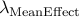• Calculated genomic-control inflation factor for heterogeneity part of RE2 statistic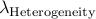How to correct for population structure

We provide a simple method for correcting for population structure for RE2.
First, put all SNPs into a single file.
Then apply our software without using -lambda_mean or -lambda_hetero options.
Look at log file to see what are the inflation factors for both the mean effect part and heterogeneity part of RE2 statistic, which we denoteand.
Then, apply our software one more time using the options -lambda_meanand -lambda_hetero.

How to correct for population structure in each single study

Often, the user wants to apply genomic control not only to the meta-analysis p-values but also to each single study before the user uses them in the meta-analysis. This can be easily done by, in the input file, increasing the standard errors by the square root of the calculated inflation factor for each study.

ForestPMPlot

Important Package Dependency :

• R : library(plotrix)
• python : numpy

Usage:

usage: python pmplot.py [meta_input] [meta_output] [study_name] [study_order] [rsid] [gene_name] [out_file]
[meta_input]                        Input file of METASOFT.
[meta_output]                       Output file of METASOFT.
[study_name]                        File containing studynames. One study name per line. The order of the
study names should be matched with [meta_input] and [meta_output] files.
[study_order]                       File containing the display index of studies. One index per line.
[rsid]                              Rsid of a SNP to generate ForestPMPlot.
[gene_name]                         Implicated gene name for the SNP.
[out_file]                          ForestPMPlot file. (Format : pdf)

Format converter

Our software converts multiple .assoc files from plink to the input format of METASOFT. If .assoc files include both A1 and A2 columns, our converter can detect simple strand flips. There are three output files: .meta file is the formatted file, .mmap file includes SNP map information, and .log file includes log information such as strand flipped SNPs, excluded SNPs, and reasons for exclusions. Python and R must be installed in the system to use our converter.

Interpretation

If an associated SNP shows high heterogeneity, interpreting the results is challenging. We provide a measure called M-value, the posterior probability that the effect exists in each study. M-values have the following interpretations.

• Small m-value (e.g. < 0.1): The study is predicted to not have an effect.

• Large m-value (e.g. > 0.9): The study is predicted to have an effect.

• Otherwise: It is ambiguous to make a prediction.

M-values can be plotted with p-values in a two-dimensional space, called PM-plot, which has the following interpretations.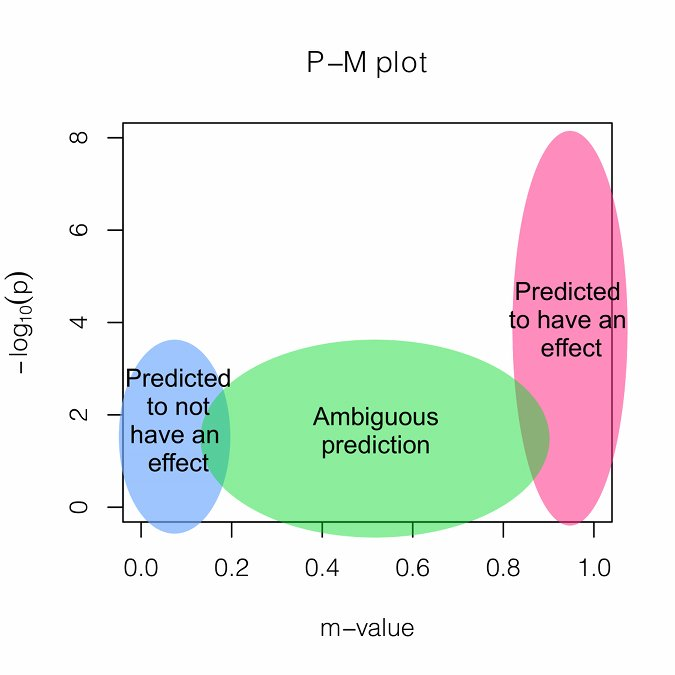GxE Interpretation

Joint analysis of multiple studies with varying environmental conditions using a meta-analysis can provide unique opportunity to identify gene-by-environment interactions. Examining both forest plot and PM-plot allows us to easily hypothesize that there is a specific group of studies showing gene-by-environment interactions. For example, let's consider study 10-11 containing mouse C57BL/6 x DBA/2 F2 strains with homozygous deficiency in leptin receptor (db/db). In forest plot, effect size estimate from male study is much larger than that of female study, but standard error of the female effect size estimates is large so that it is not clear that the effect size of female study is zero. However the m-value prediction clearly shows the distinction between male and female studies, which suggest gene-by-sex interaction under the homozygous deficiency in leptin receptor (db/db) condition.The above is an example ForestPMPlot of 17 HDL mouse studies from the paper of Kang et al.

Publication

• If you use our software or refer to the new random effects model method, please cite

Buhm Han and Eleazar Eskin, “Random-Effects Model Aimed at Discovering Associations in Meta-Analysis of Genome-wide Association Studies”, The American Journal of Human Genetics (2011) 88, 586-598. LINK

• If you use m-values or the binary effects model method, please cite

Buhm Han and Eleazar Eskin, “Interpreting Meta-Analysis of Genome-wide Association Studies”, In Press. PLoS Genetics (2012). (Manuscript available here)

• If you want to refer the GxE interpretation, please cite

Eun Yong Kang and Buhm Han and Nicholas Furlotte and Jong Wha J. Joo and Diana Shih and Richard C. Davis and Aldons J. Lusis and Eleazar Eskin, “ Meta-Analysis Identifies Gene-by-Environment Interactions as Demonstrated in a Study of 4,965 Mice ”, PLOS Genetics (2014) 10(1), e1004022. LINK

• If you use our software to plot ForestPMPlot, please cite

Eun Yong Kang and Buhm Han and Eleazar Eskin, “ ForestPMplot: a flexible tool for visualizing heterogeneity between studies in meta analysis”, Submitted

• If you refer multiple tissue eQTL method, please cite

Jae Hoon Sul and Buhm Han and Chun Ye and Ted Choi and Eleazar Eskin, “ Effectively identifying eQTLs from multiple tissues by combining mixed model and meta-analytic approaches.”, PLOS Genetics (2013) 9, e1003491. LINK Software

Contact

METASOFT

Buhm Han : buhan (AT) cs.ucla.edu , http:www.cs.ucla.du/~buhan

ForestPMPlot

Eun Yong Kang : ekang (AT) cs.ucla.edu

Funding information

B.H. E.Y.K and E.E. are supported by National Science Foundation grants 0513612, 0731455, 0729049 and 0916676, and NIH grants K25-HL080079 and U01-DA024417. B.H. is supported by the Samsung Scholarship. This research was supported in part by the University of California, Los Angeles subcontract of contract N01-ES-45530 from the National Toxicology Program and National Institute of Environmental Health Sciences to Perlegen Sciences.